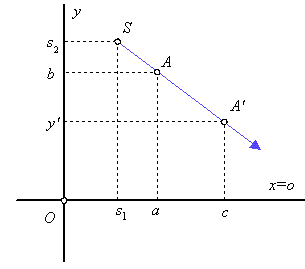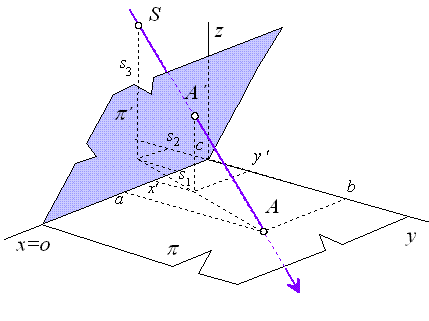# 1 Projective transformations

On the set of all points of the space ${}_{\infty }{\mathbf{E}}^{n}\left(R\right)$ with defined homogeneous coordinate system, there is defined a group of projective transformations called collineations - collinear mappings, or perspectivities. Projective transformations of the extended Euclidean space keep invariant incidence.

Ideal points of the space can be mapped to real points and real points can have their projective images at infinity. Centre of a geometric figure is not mapped to the centre of its image under the projective transformation. Size of line segments and parallelism are not invariant, therefore the size and shape of geometric figures change under these transformations.

Projective transformations of the space are: identity, central collineation, perspective collineation.

Projective transformations are analytically represented by regular square matrices of rank 4, while determinant of the matrix is nonzero, which means $|{\mathrm{T}}_{\mathrm{K}}|\ne 0$ and the matrix is in the form

 ${\mathrm{T}}_{\mathrm{K}}=\left(\begin{array}{cccc}a& b& c& p\\ d& e& f& q\\ i& j& k& r\\ l& m& n& s\end{array}\right)$

## 1.1 Projective transformations in the plane

In the two-dimensional extended Euclidean space ${}_{\infty }{\mathbf{E}}^{2}\left(R\right)$ with the defined homogeneous coordinate system, a group of projective transformations can be determined. Any point $A=$$\left(x,y,{a}_{0}\right)$ for ${a}_{0}=0,\phantom{\rule{mediummathspace}{0.2em}}1$ in the plane can be attached its image $A\text{'}=\left(x\text{'},y\text{'},{a}_{0}\right)$, while the relation of coordinates can be expressed in the following way

 $A\text{'}=A.{\mathrm{T}}_{\mathrm{K}}$

where ${\mathrm{T}}_{\mathrm{K}}\phantom{\rule{mediummathspace}{0.2em}}$is the regular square matrix of rank 3.

We recognize the following projective transformations of plane: central collineation.

### 1.1.1 Central collineation

Central collineation is transformation of the plane, which keeps point invariant one line in the plane, and one point not located on this line. A pencil of lines passing through the centre S of collineation is the set of all invariant lines (but not point invariant) under the mapping. Pairs of corresponding points in central collineation form lines of this pencil.

Let central collineation be determined by the axis of collineation in the coordinate axis x, centre $S=\left({s}_{1},{s}_{2},1\right)$ of collineation, and a pair of corresponding points A and A', while point A' must be located on line SA, $A=\left(a,\phantom{\rule{2mm}{2mm}}b,\phantom{\rule{2mm}{2mm}}1\right)↦A\text{'}=\left(c,\phantom{\rule{2mm}{2mm}}d,\phantom{\rule{2mm}{2mm}}1\right),\phantom{\rule{2mm}{2mm}}{s}_{1}\ne a,\phantom{\rule{2mm}{2mm}}b\ne 0$, thus $d=b+\left({s}_{2}-b\right)\frac{c-a}{{s}_{1}-a}$, thenFigure 1.1: Central collineation

Equations and matrix of the respective central collineation

 $x\text{'}=x+\frac{{s}_{1}\left(c-a\right)}{b\left({s}_{1}-c\right)}\phantom{\rule{2mm}{2mm}}y$
 $y\text{'}=\frac{d}{b\left({s}_{1}-c\right)}\phantom{\rule{2mm}{2mm}}y$
 ${\mathrm{T}}_{\mathrm{K}}=\left(\begin{array}{ccc}b\left({s}_{1}-c\right)& 0& 0\\ {s}_{1}\left(c-a\right)& d& c-a\\ 0& 0& b\left({s}_{1}-c\right)\end{array}\right)\approx \left(\begin{array}{ccc}1& 0& 0\\ \frac{{s}_{1}\left(c-a\right)}{b\left({s}_{1}-c\right)}& \frac{d}{b\left({s}_{1}-c\right)}& \frac{c-a}{b\left({s}_{1}-c\right)}\\ 0& 0& 1\end{array}\right)$

where

 $d={s}_{2}\left(c-a\right)+b\left({s}_{1}-c\right)$

## 1.2 Projective transformations in the space

In the three-dimensional extended Euclidean space ${}_{\infty }{\mathbf{E}}^{3}\left(R\right)$ with the defined homogeneous coordinate system, a group of projective transformations can be determined. Any point $A=$$\left(x,\phantom{\rule{2mm}{2mm}}y,\phantom{\rule{2mm}{2mm}}z,{\phantom{\rule{2mm}{2mm}}a}_{0}\right)$ for ${a}_{0}=0,\phantom{\rule{mediummathspace}{0.2em}}1$ in the plane can be attached its image $A\text{'}=\left(x\text{'},\phantom{\rule{2mm}{2mm}}y\text{'},{\phantom{\rule{2mm}{2mm}}z\text{'},\phantom{\rule{2mm}{2mm}}a}_{0}\right)$, while the relation of coordinates can be expressed in the following way

 ${A\text{'}=A.\mathrm{T}}_{\mathrm{K}}$

where ${\mathrm{T}}_{\mathrm{K}}\phantom{\rule{mediummathspace}{0.2em}}$is the regular square matrix of rank 4.

We recognize the following projective transformations of space: perspective collineation.

### 1.2.1 Perspective collineation

Perspective collineation is transformation of the space, which keeps point invariant one line in the space (axis of collineation), and one point not located on this line (called centre of collineation). A pencil of lines passing through the centre S of collineation is the set of all invariant lines (but not point invariant) under the mapping. Pairs of corresponding points in central collineation form lines of this pencil.

Let perspective collineation be determined by the axis of collineation in the coordinate axis x, centre $S=\left({s}_{1},{s}_{2},{s}_{3},1\right),\phantom{\rule{2mm}{2mm}}{s}_{3}\ne 0$ of collineation, and a pair of corresponding points $A=\left(a,\phantom{\rule{2mm}{2mm}}b,\phantom{\rule{2mm}{2mm}}0,\phantom{\rule{2mm}{2mm}}1\right)↦A\text{'}=\left(x\text{'},\phantom{\rule{2mm}{2mm}}y\text{'},\phantom{\rule{2mm}{2mm}}c,\phantom{\rule{2mm}{2mm}}1\right),\phantom{\rule{2mm}{2mm}}b\ne 0,\phantom{\rule{2mm}{2mm}}a\ne {s}_{1},\phantom{\rule{2mm}{2mm}}c\ne {s}_{3}$, while point A' must be located on line SA, thenFigure 1.2: Perspective collineation

Equations and matrix of the perspective collineation

 $x\text{'}=x+\frac{d}{b}\phantom{\rule{2mm}{2mm}}y+\frac{g}{b}\phantom{\rule{2mm}{2mm}}z$
 $y\text{'}=\frac{e}{b}\phantom{\rule{2mm}{2mm}}y+\frac{k}{b}\phantom{\rule{2mm}{2mm}}z$
 $z\text{'}=\frac{c}{b}\phantom{\rule{2mm}{2mm}}y+\frac{l}{b}\phantom{\rule{2mm}{2mm}}z$
 ${T}_{K}=\left(\begin{array}{cccc}b& 0& 0& 0\\ d& e& c& 0\\ g& k& l& 0\\ 0& 0& 0& b\end{array}\right)\approx \left(\begin{array}{cccc}1& 0& 0& 0\\ \frac{d}{b}& \frac{e}{b}& \frac{c}{b}& 0\\ \frac{g}{b}& \frac{k}{b}& \frac{l}{b}& 0\\ 0& 0& 0& 1\end{array}\right)$

where

 $d=\frac{c\left({s}_{1}-a\right)}{{s}_{3}},\phantom{\rule{2mm}{2mm}}e=b-1+\frac{c\left({s}_{2}-b\right)}{{s}_{3}},\phantom{\rule{2mm}{2mm}}g=\frac{-d{s}_{2}}{{s}_{3}}$
 $k=\frac{\left(b-e\right){s}_{2}}{{s}_{3}},\phantom{\rule{2mm}{2mm}}l=b-\frac{c\phantom{\rule{2mm}{2mm}}{s}_{2}}{{s}_{3}}$